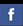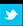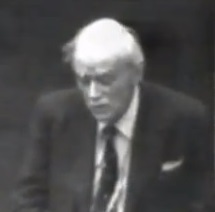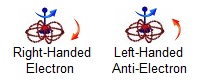Animated Physics - The mathmatical concepts of Quantum PhysicsSpinning Bloch Spheres
Larmor Frequency
Rabi Oscillations
Qantum Spin States

Protons and Neutrons
Electrons

 Paul Dirac Live!Dirac himself discussing the history of quantum mechanics and source of his discovery of the "Dirac Equation". He shows how the Poisson Bracket matches his commutators and expands on the Klien-Gordon equation for spin. Click for video feed  Summary

 Electrons as coupled 2-spinorsThese coupled spinors spin, at the speed of light, orthogonal to themselves. From the direction of spin, you can see there is no way to push the electron and anti-electron together without destroying them. Also note, turning the inner (blue) loop 360 degrees, only turns the larger (red) loop 180 degrees leaving it upside down. You must turn another 360 degrees (720 total) before it is right side up.

 Spin and Bra-ket notation From Townsend's "A Modern Approach to Quantum Mechanics", understanding spin and bra-ket notation. |ψ> (called a "ket") is an expression for a state of a system. When talking only about the intrinsic spin degree of freedom, we express the state as as |±n> where n is the axis we are measuring the spin along (ie, x, y, z or anything in between). So for example a spin 1/2 particle that is "spin up" along the z-axis could be denoted |+z> and the eigenvalue equation of it with the Sz operator would be: Sz|+z>=ℏ/2|+z> and similarly for a "spin down", Sz|−z>=−ℏ/2|−z> since |+z> and |−z> are eigenstates of the Sz operator. Derivation of the "Dirac Equation" Consider the Klien-Gordon equation (in c=hbar = 1 units) (∂²+m²)ϕ=0 You get this equation by starting with the relativistic invariant and replacing E with the energy operator and p with the momentum operator. Because this is second order, you can't reproduce the result that probability is conserved like with the Schrodinger equation (it won't hold it's normalization). So Dirac set out to find a first order equation, which would "square" to the Klien-Gordon equation, so that it would both be relativistic and preserve probability. To state the problem mathematically, you have some operator D, such that: Dψ=0 and D²ψ=(∂²+m²)ψ=0 Write down a first order operator for D, and solve for coefficients: D=(A∂−Bm), D²=(A∂−Bm)(A∂−Bm)=A²∂²+m²B²−(AB+BA)m∂=∂²+m² So A² and B² have to be one, but that doesn't allow AB + BA to be zero, as long as A and B are complex numbers. Dirac's great idea is that A and B can be matrices, and by finding a set of matrices with those properties you have derived the Dirac Equation. That is the derivation, and how it's linked to special relativity (being closely related to the Klien-Gordon equation). Anti-matter can also be predicted from solutions of the Dirac equation. Derivation of the "Dirac Electron" Dirac's one equation for a massive particle can be rewritten as two equations for two interacting massless particles, where the coupling constant of the interaction is the mass of the electron. From Penrose's Road to Reality (Chapter 25, Section 2): It turns out that the Dirac spinor ψ, with its 4 complex components, can be represented, as a pair of 2-spinors αA and βA', one with an unprimed index and one with a primed index: ψ = (αA, βA') The Dirac equation can then be written as an equation coupling these two 2-spinors, each acting as a kind of ‘source’ for the other, with a ‘coupling constant’ M describing the strength of the ‘interaction’ between the two... More formally, break an electron field into two parts (left and right) as ψ=ψL+ψR where ψL=(1/2)*(1−γ5)ψ and ψR=(1/2)*(1+γ5)ψ. These two massless fields, one left-handed and one right-handed, interact with coupling constant M equal to the mass of the electron. Learn more...